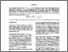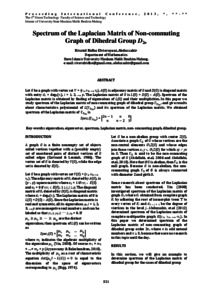# Spectrum of the Laplacian matrix of non-commuting graph of dihedral group D2n

Elvierayani, Rivatul Ridho and Abdussakir, Abdussakir (2013) Spectrum of the Laplacian matrix of non-commuting graph of dihedral group D2n. Presented at The 4th International Conference Green Technology, 9 November 2013, Faculty of Science and Technology, Maulana Malik Ibrahim State Islamic University.Preview
Text (fulltext)
1862.pdf

## Abstract

Let G be a graph with vertex set V = {v1,v2,..., vp}, A(G) is adjacency matrix of G and D(G) is diagonal matrix with entry dii = deg(vi), i = 1, 2, …, p. The Laplacian matrix of G is L(G) = D(G) – A(G). Spectrum of the Laplacian matrix is obtained by finding of eigenvalues of L(G) and their multiplicities. In this paper we study spectrum of the Laplacian matrix of non-commuting graph of dihedral group , and give results about characteristics polyniomial of L(T(D2n)) and its spectrum of the Laplacian matrix. We obtained spectrum of the Laplacian matrix of is SpecL(T(D2n))= [2n-1,n,0;n,n-2,1]

Item Type: Conference (Paper) eigenvalues, eigenvector, spectrum, Laplacian matrix, non-commuting graph, dihedral group. 01 MATHEMATICAL SCIENCES > 0101 Pure Mathematics > 010104 Combinatorics and Discrete Mathematics (excl. Physical Combinatorics) Faculty of Mathematics and Sciences > Department of Mathematics Abdussakir Abdussakir 30 Apr 2017 23:44View Item